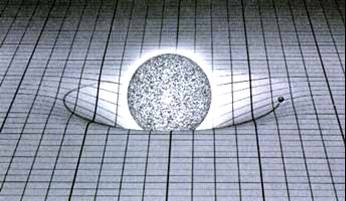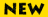Welcome to The Log of Gravity page.Reference (now with open access):

Santos Silva, J.M.C. and Tenreyro, Silvana (2006), The Log of Gravity, The Review of Economics and Statistics, 88(4), pp. 641-658.

An early version of the paper can be found at CEP/LSE (and an even earlier version at Boston Fed).

In this page you can find the data set used in the paper, codes to extend some of the results in the paper, and other useful information on the implementation of the PPML estimator.

If you cannot find here the answer to your question about The Log of Gravity, please do not hesitate to contact the authors; we will be only too pleased to help.

• The dataset (in xls and dta formats) is available here.

• Convergence problems:

1. We are aware of two situations where you may find that (at first) the PPML estimator has trouble to converge. Fortunately, there are simple solutions for both cases:
i) You may have problems using PPML when the dependent variable has many zeros and the model includes many dummies. A typical example of this is when panel data is used to estimate gravity equations including time-varying importer and exporter fixed effects. A simple explanation for why this may lead to convergence problems can be found here and this Economics Letters paper provides the more technical details. To by-pass the problem, see the ppml command below.
ii)  Stata's poisson command is not very good at dealing with numerical problems and the algorithm may not converge either if the dependent variable has very large values or if the regressors are highly collinearity or have different scales. In this case there are several possible solutions: a) you may be able to solve the problem just by re-scaling or re-centring the problematic variables; b) rather than using the poisson command you can use the ppml command that we have written (see below); c) of course, you can just use other software; one of us is a great fan of TSP.
2. Further details on convergence issues can be found in this Stata Journal paper (an earlier version is available here).
3. We have written a ppml command for Stata that bypasses most of these problems. To install the command just type "ssc install ppml"; please read the help file carefully before using the command. An updated version of the ppml command was released on 27/10/2015.4. Even using the ppml command you will have convergence problems if the base category of any of the dummy variables in the model is a prefect predictor of zero observations in the dependent variable. An example of this problem and a solution are described here.
• Incidental parameters:
Charbonneau (2012, p. 41) claimed that "a Poisson model with two fixed effects does suffer from the incidental parameter problem", and this claim has recently been echoed by other researchers. However, Charbonneau's claim is based on an example with N = T = 2, which cannot be informative about the existence of an incidental parameter problem because this problem is asymptotic in nature. In contrast, Fern�ndez-Val and Weidner (2014, example 2, p. 16) have recently proved that a Poisson model with two fixed effects does not suffer from the incidental parameter problem as long as the regressors are strictly exogenous (a requirement that is also needed for the consistency of the fixed effects estimator in liner models). Therefore, under very general conditions, inference based on the estimation by ppml of gravity equations including both importer and exporter fixed effects will not be affected by an incidental parameter problem.

• Testing competing models for non-negative data with many zeros:
We have developed a simple test to choose between competing models for non-negative data with many zeros; the paper is available here. Stata code to implement the test is available, type "ssc install hpc"; the code and data used in the paper are available here.

• Instrumental Variables:
We have written a crude Stata command (recently updated) to estimate the IV version of PPML. This estimator was originally described in Windmeijer, F. and Santos Silva, J.M.C. (1997), "Estimation of Count Data Models with Endogenous regressors: An Application to Demand for Health Care," Journal of Applied Econometrics, 12(3), pp. 281-294. Here is an example of how to use the command.
UPDATE: This estimator is now implemented in Stata 13; see the ivpoisson command and the add option (the default).

• RESET test:
Here is a sample of the code to perform the test.

• R-squared:
If you want to compute the R-squared for a model estimated by PPML, you can used the method implemented here.

• PPML performance with many zeros:
Simulation evidence on the excellent performance of the PPML estimator when the data has many zeros can be found in this Economics Letters paper.

• Warning:
Be advised that there are several papers purporting to introduce estimators that improve on the PPML. While it is of course possible to find estimators that outperform PPML in specific conditions, to our knowledge all the newly proposed alternatives to PPML are either simply invalid, or valid only under implausibly strong distributional assumptions. Therefore we stand by the claim that PPML has all the characteristics needed to be the workhorse for the estimation of constant-elasticity models such as the gravity equation. If you believe to have evidence that another estimator generally outperforms PPML in this context please do let us know; we would be delighted to acknowledge that.

• We have written a short reply to "The log of gravity revisited".

• If you want to compute 'undertrading' and 'overtrading' after fixed-effects regressions with panel data, you need to obtain a set of residuals with zero mean. Here is how to do it.

• In this Annual Review of Economics paper we use PPML and panel data to estimate the trade effect of the euro; an earlier version is available here.

• Related work by other authors:

1. James Anderson, Mario Larch, and Yoto Yotov have recently used PPML for a different purpose; their paper can be seen here.2. Thibault Fally has provided an additional motivation for using PPML; his interesting paper can be found here.
3. Paulo Guimarães has written a Stata add-on that can be useful if you want to use PPML to estimate a model with importer and exporter dummies. To install type: "ssc install poi2hdfe".
4. Our ppml command for Stata does not have an option to include country-pair fixed effects; if you really want to include them you may want to consider Timothy Simcoe's xtpqml Stata command. In recent versions of Stata you may also use xtpoisson with clustered standard errors.
• Nine FAQ's & myths about the Log of Gravity

1 - Why can't we just use the log-linear model with robust/clustered standard errors?
2 - Does PPML perform poorly when the data has a large proportion of zeros?
3 - Does PPML assume equi-dispersion?
4 - How can a continuous variable like exports have a Poisson distribution?
5 - Why use a count data estimator when the dependent variable is continuous?
6 - Why not use other count data models like the negative-binomial or zero inflated models?
7 - Can Vuong's tests for non-nested hypotheses be used to choose between the PPML and the ZIP?
8 - Can we use the AIC and BIC to choose between PPML and other methods or models?
9 - Can PPML be used when there is over-dispersion in the data?

According to Web-Counter, there have beenunique visitors to this page since 15 December 2008

Last updated on 11 November 2015